# Python Tensor FLow简单使用方法实例详解_python

pip是Python的包管理器，在新的发行版本中会默认安装在命令行下输入pip list比较老的版本可能需要输入pip freeze即可显示所有的安装包www.zgxue.com防采集请勿采集本网。

## 1、基础概念

Python 其实挺简单的，也挺强大的。我用 Python 做科学计算，自学一年，也记了一年的笔记。笔记链接： GitHub-中文 Python 笔记 Jupyter Notebook-中文 Python 笔记 笔记目录： 01.Python 工具 01.01Tensor表示张量，是一种多维数组的数据结构。Flow代表流，是指张量之间通过计算而转换的过程。TensorFLow通过一个计算图的形式表示编程过程，数据在每个节点之间流动，经过节点加工之后流向下一个节点。

import theano.tensor as T，import就是导入或者引用的意思了。这句话是引用类 theano.tensor 并简化为T使用，后面的T.lscalar()实际就是theano.tensor.lscalar() index=T.lscalar()，x=T.matrixUbuntu安装tensorflow 先安装python-dev，再安装tensorflow就好了 sudo apt-get install python-dev pip install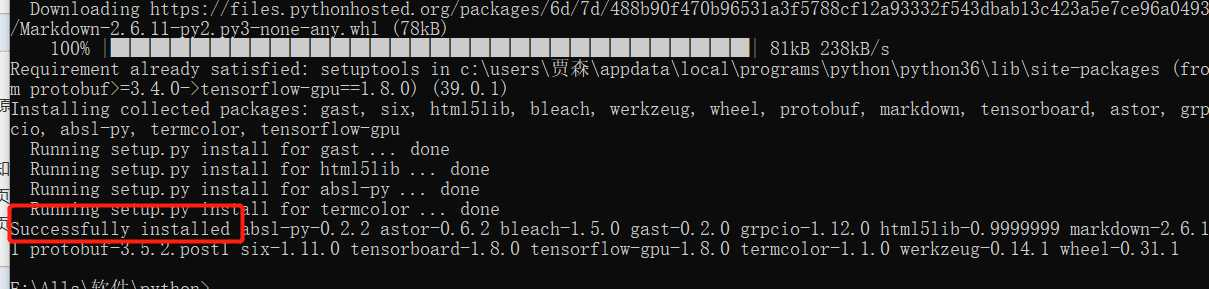import tensorflow as tf node1=tf.constant(3.0,tf.float32,name='node1') #创建浮点数节点node2=tf.constant(4.0,tf.float32,name='node2')node3=tf.add(node1,node2) #节点三进行相加操作,源于节点1、2ses=tf.Session()print(node3) #输出张量：Tensor("Add_3:0", shape=(), dtype=float32)print(ses.run(node3)) #通过会话运行节点三，将节点1、2相加，输出：7.0ses.close() #不使用时，关闭会话

tensor1=tf.constant([[[1,1,1],[1,2,1]], [[2,1,1],[2,2,1]], [[3,1,1],[3,2,1]], [[4,1,1],[4,2,1]]],name='tensor1')print(tensor1)ss=tf.Session()print(ss.run(tensor1)[3,0,0]) #访问tensor1的具体元素#输出：Tensor("tensor1:0", shape=(4, 2, 3), dtype=int32) 4

## 2、运算

### 会话

#定义计算图tensor1=tf.constant([1,2,3])#创建会话ss=tf.Session()#利用会话进行计算操作print(ss.run(tensor1))#关闭会话ss.close()

tensor1=tf.constant([1,2,3])with tf.Session() as ss: #上下文管理器 print(ss.run(tensor1))

tensor1=tf.constant([1,2,3])ss=tf.Session()with ss.as_default(): #指定默认会话 print(tensor1.eval())

tensor1=tf.constant([1,2,3])ss=tf.InteractiveSession() #自动注册默认会话print(tensor1.eval())ss.close()

### 变量、常量

TensorFLow通过constant函数完成对常量的定义，可以为其赋初值与命名

a=tf.constant(10,'int_a')

node1=tf.Variable(3.0,name='node1') #定义变量node2=tf.Variable(4.0,name='node2')res=tf.add(node1,node2,name='res') ss=tf.Session() init=tf.global_variables_initializer() #定义全部变量的初始化操作ss.run(init) #执行初始化操作 print(ss.run(res))ss.close()

TensorFLow的变量一般不需要手动赋值，因为系统会在训练过程中自动调整。如果不希望由模型自动赋值，可以在定义时指定属性trainable=False，并通过assign函数来手动赋值

var1=tf.Variable(0,name='var')one=tf.constant(1)var2=tf.add(var1,one) #变量1加1得到变量2update=tf.assign(var1,var2) #定义update操作，将变量2赋值给变量1 init=tf.global_variables_initializer()ss=tf.Session()ss.run(init)for _ in range(10): ss.run(update) #执行update操作 print(ss.run(var1)) ss.close() #输出：1 2 3 4 5 6 7 8 9 10

### 占位符

node1=tf.placeholder(tf.float32,name='node1') #定义占位符，规定其类型、结构、名字node2=tf.placeholder(tf.float32,name='node2') m=tf.multiply(node1,node2,'multinode') ss=tf.Session()res=ss.run(m,feed_dict={node1:1.2,node2:3.4}) #在运行时通过feed_dict为占位符赋值 print(res)ss.close()

node1=tf.placeholder(tf.float32,,name='node1') #第二个参数规定占位符的类型为3维数组node2=tf.placeholder(tf.float32,,name='node2') m=tf.multiply(node1,node2,'multinode')s=tf.subtract(node1,node2,'subnode') ss=tf.Session()#将m，s两个操作放到一起，并返回两个结果resm,ress=ss.run([m,s],feed_dict={node1:[1.0,2.0,4.0],node2:[3.0,5.0,6.0]})print(resm) #输出：[ 3. 10. 24.]ss.close()

## 3、TensorBoard

TensorBoard是TensorFLow的可视化工具，通过程序运行中输出的日志文件可视化地表示TensorFLow的运行状态。其编程如下：

node1=tf.Variable(3.0,name='node1') node2=tf.Variable(4.0,name='node2')res=tf.add(node1,node2,name='res')ss=tf.Session() init=tf.global_variables_initializer() ss.run(init) print(ss.run(res))ss.close() #清除default graph和其他节点tf.reset_default_graph()#定义日志存放的默认路径logdir='D:\Temp\TensorLog'#生成writer将当前的计算图写入日志writer=tf.summary.FileWriter(logdir,tf.get_default_graph())writer.close()

TensorBoard已经随Anaconda安装完成，首先通过Anaconda Prompt进入日志文件的存放目录，然后输入tensorboard --logdir=D:\Temp\TensorLog，设定日志的存放路径，完成之后在浏览器的localhost:6006端口就可以看到TensorBoard，也可以通过--port命令修改默认端口。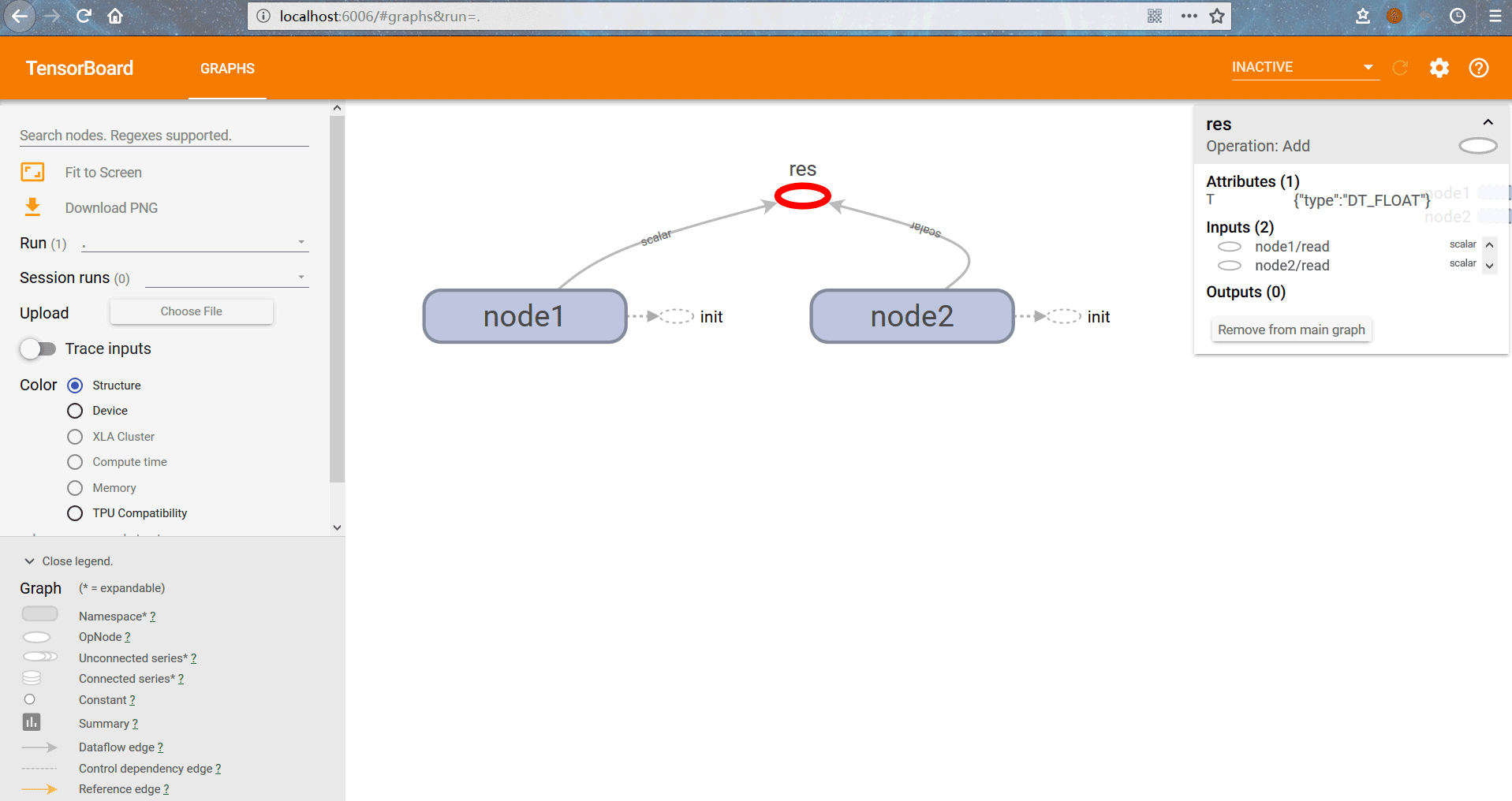#TensorBoard使用#定义日志保存位置log_dir='D:\Temp\MachineLearning\TensorLog'#显示图片image_input=tf.reshape(x,[-1,28,28,1])tf.summary.image('input',image_input,10) #一次最多显示图片数：10#显示直方图tf.summary.histogram('Y',Y3)#显示标量losstf.summary.scalar('loss',loss_function)tf.summary.scalar('accurancy',accuracy)#定义汇总summary操作merge_op=tf.summary.merge_all() ss=tf.Session()ss.run(tf.global_variables_initializer())#定义writerwriter=tf.summary.FileWriter(log_dir,ss.graph) for epoch in range(train_epochs): for batch in range(batch_num): #分批次读取数据进行训练 xs,ys=mnist.train.next_batch(batch_size) ss.run(optimizer,feed_dict={x:xs,y:ys}) #执行summary操作并将结果写入日志文件 summary_str=ss.run(merge_op,feed_dict={x:xs,y:ys}) writer.add_summary(summary_str,epoch) loss,acc=ss.run([loss_function,accuracy],\ feed_dict={x:mnist.validation.images,y:mnist.validation.labels}) print('第%2d轮训练：损失为：%9f，准确率：%.4f'%(epoch+1,loss,acc))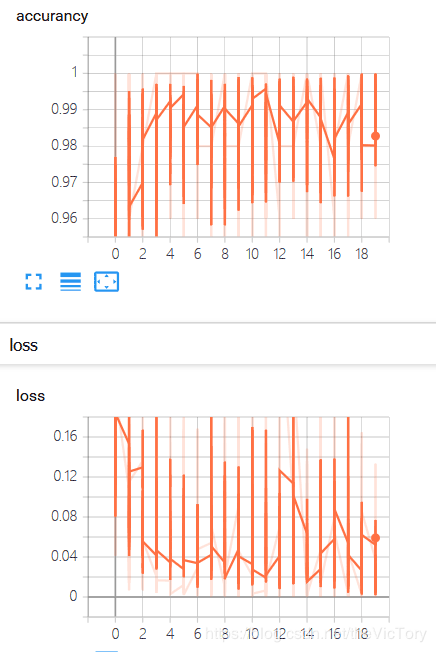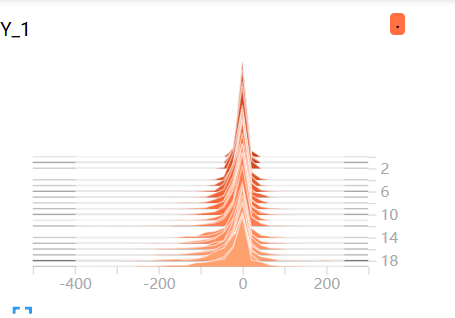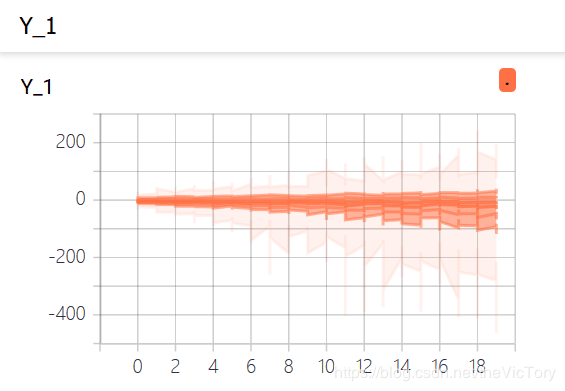tensorflow网站上给出了新的使用Anaconda配置和安装Tensorflow的步骤，经过测试，在国内可以无障碍的访问。Anaconda 是一个基于Python的科学计算包集合，目前支持Python 2.7和3.5内容来自www.zgxue.com请勿采集。

• 本文相关：
• tensorflow查看输入节点和输出节点名称方式
• tensorflow查看ckpt各节点名称实例
• 如何定义tensorflow输入节点
• python实现从订阅源下载图片的方法
• python def函数的定义、使用及参数传递实现代码
• python3 socket同步通信简单示例
• 跟老齐学python之有容乃大的list(4)
• python 从远程服务器下载日志文件的程序
• python实现一个简单的并查集的示例代码
• 让你python到很爽的加速递归函数的装饰器
• python实现两张图片的像素融合
• python抓取网页内容示例分享
• 和孩子一起学习python之变量命名规则
• Python 中有计算tensor 的包吗
• Python 中有计算tensor 的包吗
• Python 中有计算tensor 的包吗
• pytho 怎么安装tensor
• 如何系统地自学 Python
• 关于python代码的问题
• tensor flow 用什么方式安装好
• python中怎么删除数组的每一个元素的首字母？
• python 计算程序运行了多长时间
• 如何系统地自学 Python
• 免责声明 - 关于我们 - 联系我们 - 广告联系 - 友情链接 - 帮助中心 - 频道导航